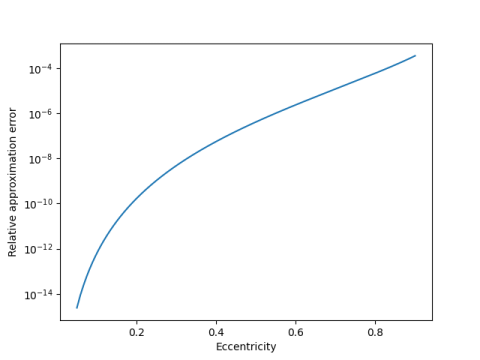# Simple approximation for perimeter of an ellipse

The perimeter of an ellipse cannot be computed in closed form. That is, no finite combination of elementary functions will give you the exact value. But we will present a simple approximation that is remarkably accurate.

So this post has two parts: exact calculation, and simple approximation.

## Exact perimeter

The perimeter can be computed exactly in terms of an elliptic integral. The name’s not a coincidence: elliptic integrals are so named because they were motivated by trying to find the perimeter of an ellipse.

Let a be the length of the major semi-axis and b the length of the minor semi-major axis. So a > b, and if our ellipse is actually a circle, a = b = r.

If ε is the eccentricity of our ellipse,

ε² = 1 – b² / a²

and the perimeter is give by

p = 4a E(ε²)

where E is the “complete elliptic integral of the second kind.” This function has popped up several times on this blog, most recently in the post about why serpentine walls save bricks.

You could calculate the perimeter of an ellipse in Python as follows.

    from scipy.special import ellipe

def perimeter(a, b):
assert(a > b > 0)
return 4*a*ellipe(1 - (b/a)**2)


## Simple approximation

But what if you don’t have a scientific library like SciPy at hand? Is there a simple approximation to the perimeter?

In fact there is. In  the authors present the approximationIf we define the effective radius as r = p/2π, then the approximation above saysin the notation of Hardy, Littlewood, and Pólya, where x = (a, b).

We could code this up in Python as follows.

    def rmean(r, x):
n = len(x)
return (sum(t**r for t in x)/n)**(1/r)

def approx(a, b):
return 2*pi*rmean(1.5, (a, b))


It would have been less code to write up approx directly without defining rmean, but we’ll use rmean again in the next post.

For eccentricity less than 0.05, the error is less than 10-15, i.e. the approximation is correct to all the precision in a double precision floating point number. Even for fairly large eccentricity, the approximation is remarkably good.The eccentricity of Pluto’s orbit is approximately 0.25. So the approximation above could compute the perimeter of Pluto’s orbit to nine significant figures, if the orbit were exactly an ellipse. A bigger source of error would be the failure of the orbit to be perfectly elliptical.

If an ellipse has major axes several times longer than its minor axis, the approximation here is less accurate, but still perhaps good enough, depending on the use. There is an approximation due to Ramanujan that works well even for much more eccentric ellipses, though it’s a little more complicated.

To my mind, the most interesting thing here is the connection to r-norms. I’ll explore that more in the next post, applying r-norms to the surface area of an ellipsoid.

 Carl E. Linderholm and Arthur C. Segal. An Overlooked Series for the Elliptic Perimeter. Mathematics Magazine, June 1995, pp. 216-220

## 3 thoughts on “Simple approximation for perimeter of an ellipse”

1. Jan Van lent

He was particularly interested in approximations involving only rational numbers.

The following formula is rational (no irrational constants and no square roots) and gives an approximation with a relative error less than 0.0065 for any values of a and b.
u = (a-b)/(a+b),
P = (a+b) * (22/7 + (4-22/7)*u**2)
Replacing 22/7 by a better approximation for pi gives a better approximation for nearly circular ellipses (u near 0), but it does not make a difference for the overall error.

An even better approximation (relative error less than 0.00175) is
P = (a+b) * (22/7 + 11/14*u**2 + 1/14*u**4)

The approximations are obtained by writing the ellipse perimeter as (a+b) * f(u) and approximating f(u) by fitting values (and derivates) at u=0 and values at |u|=1.
The value of pi is replaced by a rational that does not impact the overall relative error too much.

 Why is there no equation for the perimeter of an ellipse‽, Stand-up Maths, 5 Sept 2020

2. Laphm Lamph

If major axis a = 1 and minor axis is 0 < b < 1 then
P ~= (1+b) * (267/85 + 95/119 * ((1-b)/(1+b))**2 + 24/394 * ((1-b)/(1+b))**6

3. Maher Ezzeddin Aldaher

From:Maher Ezzeddin Aldaher
This my eq. In general practical form n=1 to infinity.
The formula for calculating the perimeter of a superellipse is given by:

P = 4aE(n/2,m)

Where a is the length of half of the major axis, n is the order of the superellipse, m is the curvature factor, and E(n/2,m) is the elliptic integral of the second kind with parameters n/2 and m.

This formula applies to superellipses that are symmetric about both the x and y axes. For asymmetric superellipses, the perimeter can be calculated by numerical integration or approximation methods.
P=4[a+b*(((2.5/(n+0.5))^(1/n))*b+a*(n-1)*0.566/n^2)/(b+a*(4.5/(0.5+n^2)))]
Which the research presented at ICMS2012 Nagpur- India